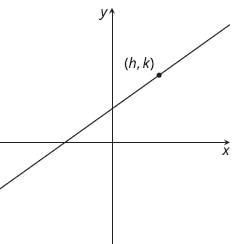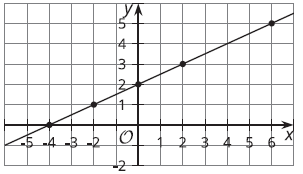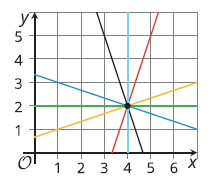# A.6.9.3 Using Point-Slope Form

The point-slope form of the equation of a line is y - k = m(x - h) where (h, k) is a particular point on the line and m is the slope of the line. (Definition)Write an equation that describes each line. a. the line passing through point (-2, 8) with slope 4/5 b. the line passing through point (0, 7) with slope -7/3 c. the line passing through point (1/2, 0) with slope -1 d. the line in the image:Using the structure of the equation, what point do you know each line passes through? What’s the line’s slope? a. y - 5 = 3/2(x + 4) b. y + 2 = 5x c. y = -2(x - 5/8)Here are the equations for all of the lines above: y = 2 x = 4 y - 2 = 1/3(x - 4) y - 2 = -1/3(x - 4) y - 2 = -3(x - 4) y - 2 = 3(x - 4) What do you notice?

Consider the line represented by y + 4 = 2/5(x - 9). Write an equation representing a different line with the same slope that passes through the point (3, 6).

Consider the line represented by y + 4 = 2/5(x - 9). Write an equation representing a different line that passes through the same point but has a slope of -3.